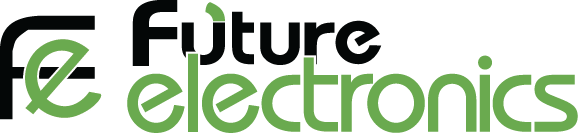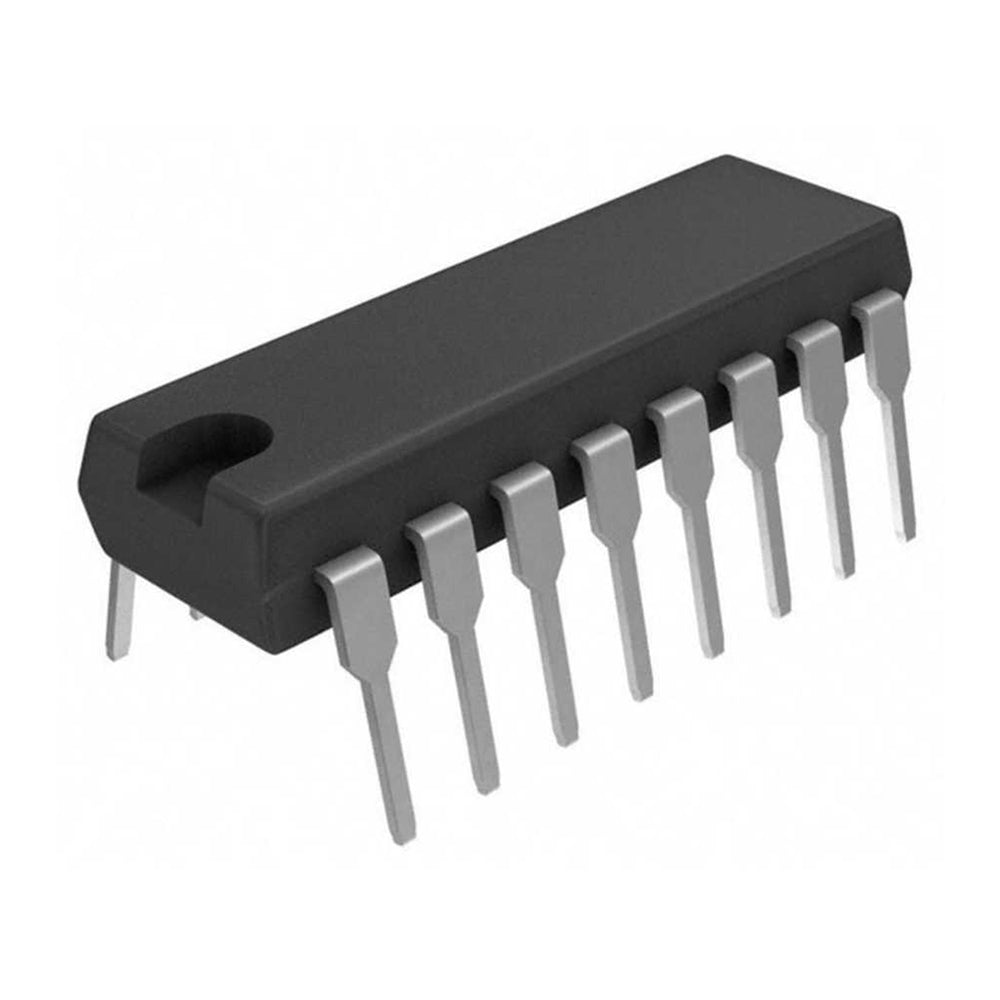1

#### Future Electronics Egypt# 74HC283 ( 4 Bit Binary Adder)

## LE 25.00

The 74HC283 adds two 4-bit binary words (An plus Bn) plus the incoming carry (CIN).

The binary sum appears on the sum outputs (S1 to S4) and the out-going carry (COUT) according to the equation:

CIN + (A1 + B1) + 2(A2 + B2) + 4(A3 + B3) + 8(A4 + B4)

= S1 + 2S2 + 4S3 + 8S4 + 16COUT

Where (+) = plus.

Due to the symmetry of the binary add function, the 74HC283 can be used with either all active HIGH operands (positive logic) or all active LOW operands (negative logic).

In case of all active LOW operands the results S1 to S4 and COUT should be interpreted also as active LOW. With active HIGH inputs, CIN must be held LOW when no carry in is intended. Interchanging inputs of equal weight does not affect the operation, thus CIN, A1, B1 can be assigned arbitrarily to pins 5, 6, 7, etc.

74HC283 datasheet

Sale

Unavailable

Sold Out